# Centripetal Force

## Laws Of Motion of Class 11

If a particle moves with speed v in a circle of radius r, it has an acceleration of magnitude v2/r directed toward the centre of the circle. This centripetal acceleration is related to the change in the direction of the velocity of the particle. As with any acceleration, there must be a resultant force in the direction of the acceleration to produce it. This resultant force is called the centripetal force. It is important to understand that the centripetal force is not a new basic interaction. It is merely a name for the resultant inward force that must be present to provide the centripetal acceleration needed for circular motion. The centripetal force may be due to a string, spring or contact force such as a normal force or friction; it may be an action−at −a distance type of force such as a gravitational force or an electrostatic force; or it may be a combination of any of these familiar forces.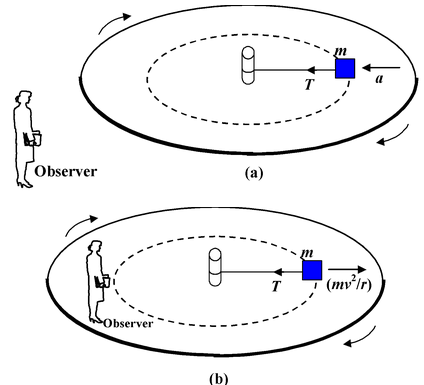Fig.(6.30)A block is tied to the centre post of a rotating platform by a string. (a)An inertial observer sees the block moving in a circle with centripetal acceleration provided by the unbalanced force T. (b)According to a non inertial observer on the platform, the block is not accelerating. Newton’s second law can be used only if a pseudo force mv2/r acting outward is introduced, to balance the tension T.

Example: 6.18

A particle of mass m is suspended from a string of length L and travels at a constant speed
v in a horizontal circle of radius r. The string makes an angle θ given by sinθ = r/L, as shown in figure. Find the tension in the string and the speed of particle.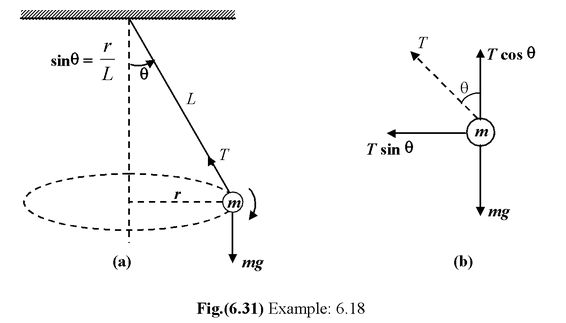Solution

The two forces acting on the particle are that due to the earth’s attraction mg, acting vertically downward, and the tension T, which acts along the string. In this problem we know that the acceleration is horizontal, towards the centre of the circle, and of magnitude v2/r thus the vertical component of the tension must balance the weight mg. The horizontal component of the tension is the resultant centripetal force. The vertical and horizontal components of ΣF = ma, therefore give

T cos θ − mg = 0

T sin θ =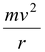The tension is found directly from the first equation since θ is given. We can find the speed v in terms of the known quantities r and θ by dividing one equation by the other to eliminate T. we obtain

tanθ =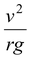or v = √rg tan θ

 Example: 6.19 A bucket of water is whirled in a vertical circle of radius r. If its speed is v at the top of the circle, find the force exerted on the water by the bucket. Find also minimum value of v for the water to remain in the bucket. Solution The forces on the water at the top of the circle are shown in figure. They are the force of gravity mg and force N exerted by the bucket. Both these forces act downward.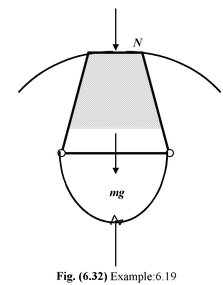The acceleration, which is toward the center of the circle, is also downward at this point. Newton’s second law gives.

N + mg =The force exerted by the bucket is therefore,− mg = N

Note that both the force of gravity and the contact normal force exerted by the bucket contribute to the necessary centripetal force.,

If we increase the speed the bottom of the bucket will exert a large force on the water to keep it moving in a circle. If we decrease the speed, N will decrease. Since the bucket cannot exert an upward force on the water, the minimum speed the water can have at the top of the circle occurs when N = 0. Then

mg =min

orvmin = √rg

When the water is moving at this minimum speed, its acceleration at the top of the path is g, the free−fall acceleration of gravity, and the only force acting on the water is the gravitational attraction of the earth, i.e. weight mg.

Example: 6.20

A small coin is placed at the rim of a turntable of radius 15 cm which rotates at 30 rev/min. Find the minimum coefficient of friction for the coin to stay on.

Solution

The free body diagram of the coin is shown in Fig. (6.33) The acceleration is toward the center so we choose the +x axis in this direction. The necessary centripetal force is provided by the friction force f. This is static friction since the coin does not slip on the table.

 Applying Newton’s Second Law ΣFx = max ⇒ f =ΣFy = may ⇒ N − mg = 0 Since f = µsN = µs(mg), we find µs mg =or µs =Here ω = 2πn/60 = πn/30 r = 0.15 m andv = rω ∴ μs =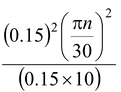= 0.148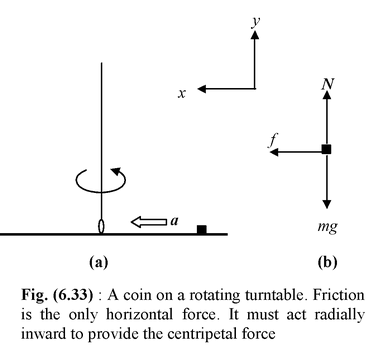Example: 6.21

In a carnival ride called the rotor, people stand on a ledge inside a large cylinder that rotates about a vertical axis. When it reaches a high enough rotational speed, the ledge drops away. Find the minimum coefficient of friction for the people not to slide down. Take the radius to be 2 m and the period of rotation to be 2 s.

 Solution The forces acting on a person is shown in the figure. Applying Newton’s Second Law f = mg(i) N = mω2R(ii) Since f = µsN ∴ µs =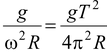Here T = 2 s; R = 2 m; g = 10 m/s2 ∴ µs = 0.5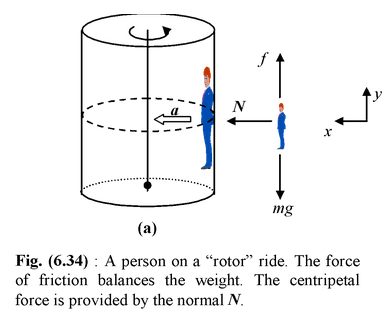Example 6.22

On fast highways or indoor bicycle tracks the outside edge of curves is raised. Such banking prevents the vehicle from slipping sideways if there is not enough friction to provide the centripetal force. A car of mass 1000 kg rounds a circular curve of radius 10 m that is banked at 37° to the horizontal. The road is slippery, so the coefficient of static friction is only 0.1. Find the maximum safe speed at which the car can travel.

Solution

We want the car to travel in a horizontal circle which means its acceleration is also horizontal. Since it is helping to prevent sliding up the incline, toward the outer edge of the circle, the frictional force must point down the incline.

From the free−body diagram in figure (b) we see that its components are

 ΣFx = N sin θ + f cos θ =(i) ΣFy = N cos θ − f sin θ − mg = 0(ii) With f = µsN = 0.1 N, cos 37° = 0.8 and sin 37° = 0.6 vmax = 8.89 m/s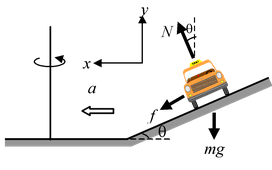Fig.6.35 (a)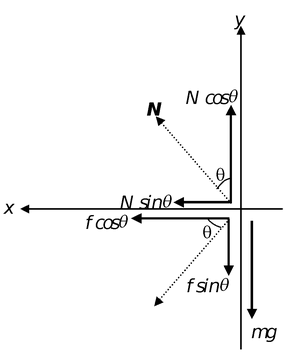Fig.6.35 (b)